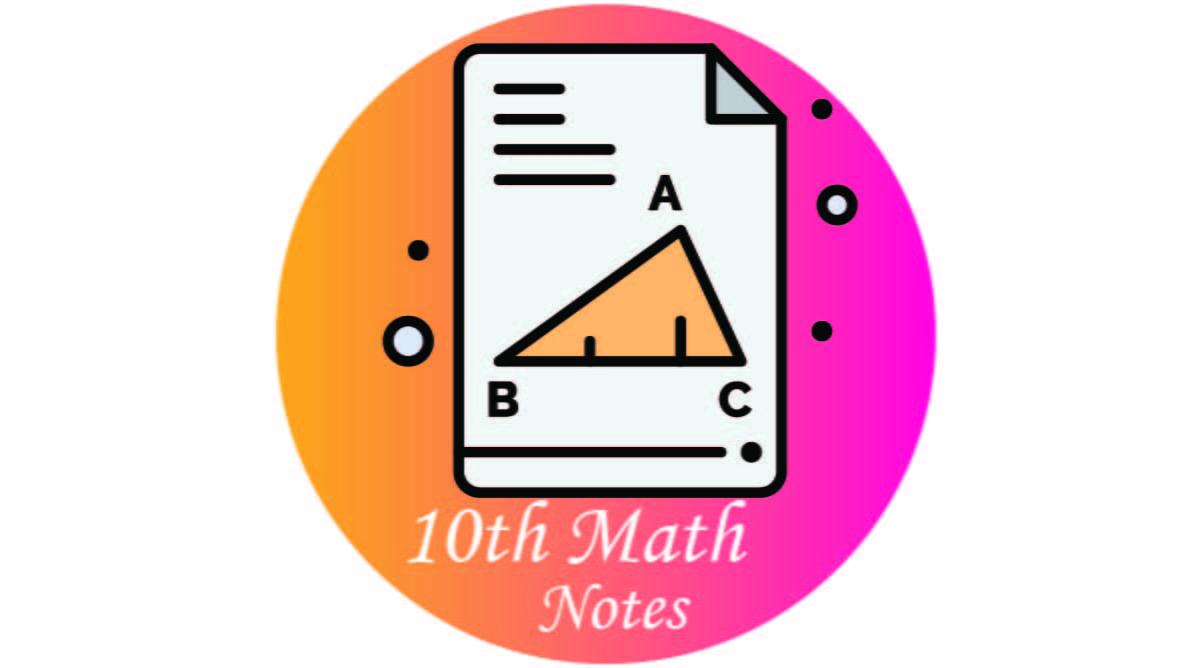# 10th Class Math Notes

0
63## 10th Class Math Chapter Wise Short Questions Definitions and MCQs Notes. These notes are very well designed, which will be of great benefit to you. With our educational materials, You can get a hundred percent score by preparing these notes. This educational material has been overseen by experienced teachers of mathematics.

There are 13 chapters of mathematics. Be sure to read it very well explored. What are the things given in all the arithmetic matrices are very well described?

## 4- Math Only Definitions

Unit 1: Algebraic Formula and Applications.

Algebraic Expression, Rational Expression, Proper Rational Expression, Improper Rational Expression, examine a Given Algebraic Expression, Rational Expression in Its Lowest Terms, Reduce a Rational Expression to Its Lowest Terms, Sum, Difference and Product of Rational Expressions, Division of a Rational Expression, Value of an Algebraic. Surds and Their Application, Surds of Second Order, Rationalizing The Denominator, Rationalization, Rationalization of Surds, Ex 1.3, Review Ex 1 and Summery

Unit 2: Factorization

Factorization of Expressions, Linear Polynomial, Quadratic Polynomials, Cubic Polynomials, Ex 2.1, Ex 2.2, Ex 2.3. Remainder Theorem and Factor Theorem, The Remainder Theorem, Finding Remainder without Dividing, zero of a Polynomial, The Factor Theorem, Factorizing a Cubic Polynomial, Ex 2.4, Ex 2.5, Review Ex 2 and Summery

Unit 3: Algebraic Manipulation

Highest Common Multiple (H.C.M) and Least Common Multiple (L.C.M), HCF, Ex 3.1, H.C.F by Division Method, Ex 3.2. Least Common Multiple (L.C.M), LCM by Factorization, Ex 3.3, Relationship between HCF and LCM, Ex 3.4 Q(1-8). Ex (3.4) Q ( 9-17), Basic Operations on the Algebraic Fractions, Addition and Subtraction of the Algebraic Fractions ,Multiplication and Division of the Algebraic  Fractions, Ex 3.5. Square root of an Algebraic Expression, Square root by Factorization Method, Square Root by Division Method, Ex 3.6, Review 3, and Summery.

Unit 4: Linear Equations and Inequalities

Linear Equations, Linear Equation in One Variable, Solution of a Linear Equation, Equations involving Radicals, Ex 4.1 Equation involving Absolute Value, Linear Inequalities, Properties of Inequalities, Solving Linear Inequalities, Ex 4.2, Review Ex 4 and Summery. Quadratic Equations, Solution of a Quadratic Equation, Solution of a Quadratic Equation, Solution of a Quadratic Equation by Factorization, Solution of a Quadratic Equation by Completing the Square Method, Ex 5.1, The Quadratic Formula, Derivation of Quadratic formula, Ex 5.2, Q(1-6)

Unit 6: Matrices and Determinants

Introduction, Order of a Matrix, Matrix Equality, Ex 6.1, Types of Matrices, Ex 6.2, Addition and Subtraction of Matrices, Laws of Addition of Matrices, Additive Identity of matrices, Additive Inverse of a Matrix, Ex 6.3. Multiplication of Matrices, Associative Law of Matrices with respect to Multiplication, Distributive Laws, Commutative Law, Theorem, Ex 6.4, Multiplicative Inverse of a Matrix, Evaluate Determinant of a Matrix, Singular and Non-Singular. Matrices, Adjoin of a Matrix, Multiplicative Inverse, Inverse of a Non-Singular Matrix,  Verify =, Ex 6.5 Q (1-3).

Unit 7: Fundamentals of Geometry

Properties of Angles, Adjacent, Complementary And Supplementary Angles, Vertical angles, Calculate Unknown Angles, Calculate Unknown Angles of a Triangle, Ex 7.1, Parallel Lines, Properties of Parallel Lines, Transversal, Relation Between the Pair of Angles, Ex 7.2 Congruent And Similar Figures, Symbol(, Properties of Congruency, Ex 7.3, Congruent Triangles, Ex 7.4, Quadrilaterals, Properties of Congruency, Opposite Side of a Rectangle are Equal, Properties of Parallelogram, Ex 7.5. Circle, Centre, Radial Segment, Radius, Chord, Segment of a Circle, Diameter, Arc, Sector, Secant Line, Tangent, Noncyclic Points, Concentric Circles, Properties of Angles, Angel in Segment, Angle in a Semi-Circle is a Right Angle, Angles in the Same Segment are Equal, Central Angle, Applications, Ex 7.6, Review 7 and Summery

Unit 8: Practical Geometry

Construction of a Triangle, Angle Bisector of a Triangle, Perpendicular Bisector of The Sides of a Triangle, Ex 8.1 Q(1-8) Construction of Quadrilateral, Rectangle, Square, Parallelogram, Ex 8.1 Q (9-19). Tangent to The Circle, Locate the center of the circle, Draw a circle Passing thorough three non – Collinear Points, Tangent to a Circle, Drawing Tangent two Equal Circles, Drawing Tangents Two Unequal Circles, Drawing Tangent Two Unequal Touching Circles, Ex 8.1, Q( 20-28), Review EX 8 and Summery.

This site uses Akismet to reduce spam. Learn how your comment data is processed.